# Summary Value - Top level not defined in listHi,

I have requirement in which I need the Total Cash value - which is kind of top level but the top level is not defined in the list configuration so I have tried my best to get the Total cash value for the "Sweep In" checks but unable to get the value without using the select statement as I have to make this list as production list

So I would like to know how to get the Total cash value without using the select statement. Screenshot attached for Reference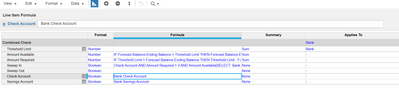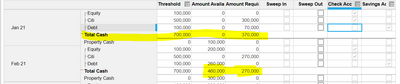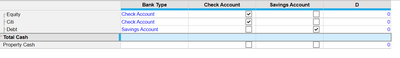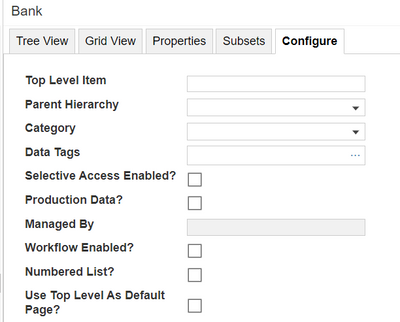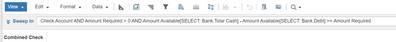So I want to get the Total cash value and subtract that with Debt Bank value

Regards,

Riyaz Pasha

Regards,

Riyaz Pasha

Tagged:

•Hi

Summary function works as a sum of the leaf elemtnts of the list. To subtract something you have to select one of two options:

- ask users to enter negative value. Then automatic sum will work correctly, like: 2 + -1 = 1

- add a calculation line item wich will multiply by -1 those list elements which you want to be negative and then use the summary from this line item as a calculated value. (for this you would have to make more additional steps such as usin Ratio summary function). Let me know if you want to go this way - I will explain.

The second method is a bit more complicated than the first one and I think you should use it only if you can't make user enter the minus sign.

•Thanks for the prompt response,

The users cannot enter negative value as those will be the bank balances.

So I would like to go with the second approach. Basically I need the Total cash value for that day/month and remove the Debt value(which means subtract saving accounts value) to compare the other line items

Regards,

Riyaz Pasha

Regards,

Riyaz Pasha

•1) in calc put a formula: threshold limit * IF Savings Account THEN -1 ELSE 1

2) in '1' just = 1  (summary method: Max)

3) select threshold limit and change Summary method to Ratio. with the following settings: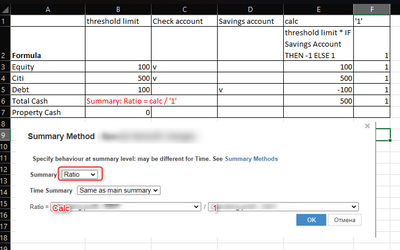•Thanks for the solution.

But Can I replace the select part with some other alternative solution as I have to show the user from which Bank they can sweep in from and Sweep out to. So in order for that I was getting the value of Amount Available for total Cash subtract with Debt Bank which will give me the other banks value where I have to check if that greater than the Amount Required so I want the select to be replaced with some alternative solution as I need to make the Bank list a Production list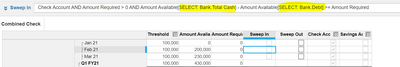Thank you,

Regards,

Riyaz Pasha

Regards,

Riyaz Pasha

•@riyazpasha

You can. General rule is that you avoid using select function anywhere except Top level elements of the lists and versions. Instead you can use LOOKUP. You can make a system module without any dimension and place any list formatted line item here for reference via lookup. For example, "Total Cash" line item with Total cash element selected manually in it.

•Hi Riyaz @riyazpasha ,

1. Create 2 line items Total Cash & Debt in the same module without any dimension.

2. Create a System module with 2 line items Total Cash 1 & Debt 1 having BANK List format and without any dimension. Manually Map Total Cash & Debt.

3. Go to the source module and formulate:

Total Cash - Treshhold[LOOKUP: System Test.'Total Cash 1']

Debt - Treshhold[LOOKUP: System Test.'Debt 1']

Refer the screen shots for more undersanding.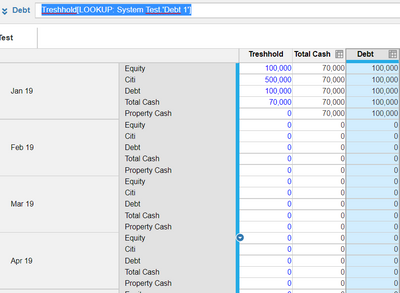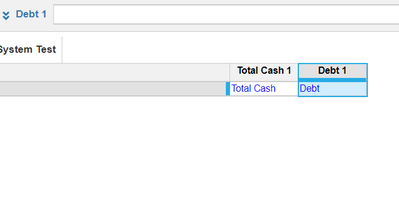Hope this helps!

Thanks,

Arnab